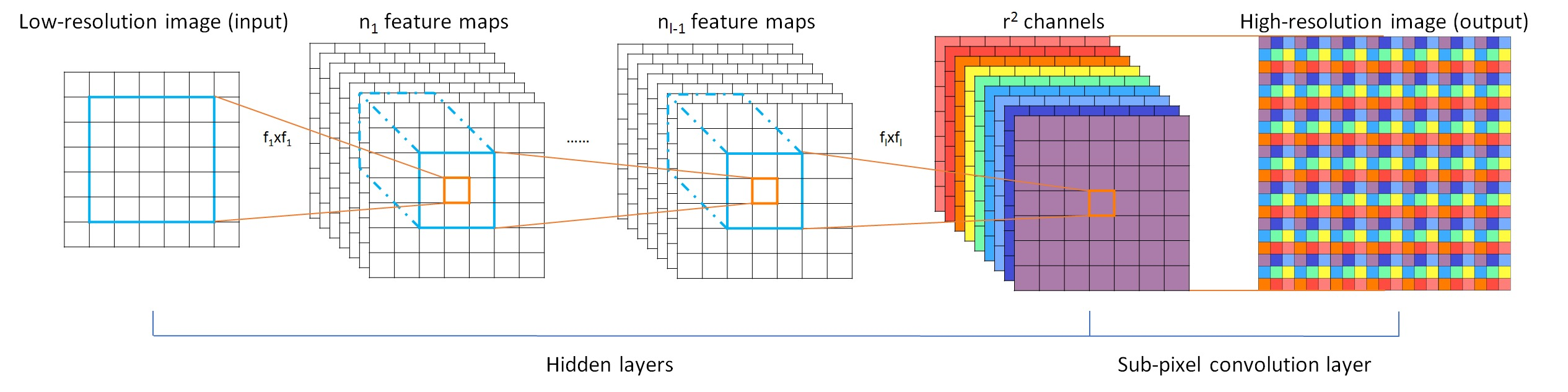# Efficient Sub-Pixel Convolutional neural Network (ESPCN)Figure 1. The proposed efficient sub-pixel convolutional neural network (ESPCN), with two convolution layers for feature maps extraction, and a sub-pixel convolution layer that aggregates the feature maps from LR space and builds the SR image in a single step.

## Contrast to previous works

• super-resolve only at the end of the network (efficient sub-pixel convolution layer) → Eliminate the need to perform super-resolve in high resolution

## Efficient sub-pixel convolution layer

• Last layer of ESPCN
• Rearrange tensor of $H \times W \times C \cdot r^2$ to $rH \times rW \times C$ tensor like Figure 1.
• Implemented at TensorFlow as depth to space and PyTorch as PixelShuffle

• Learnable $n$ upscaling filters
• Network learn $n$ upscaling filters for feature maps at last layer rather than one upscaling filter for input image.